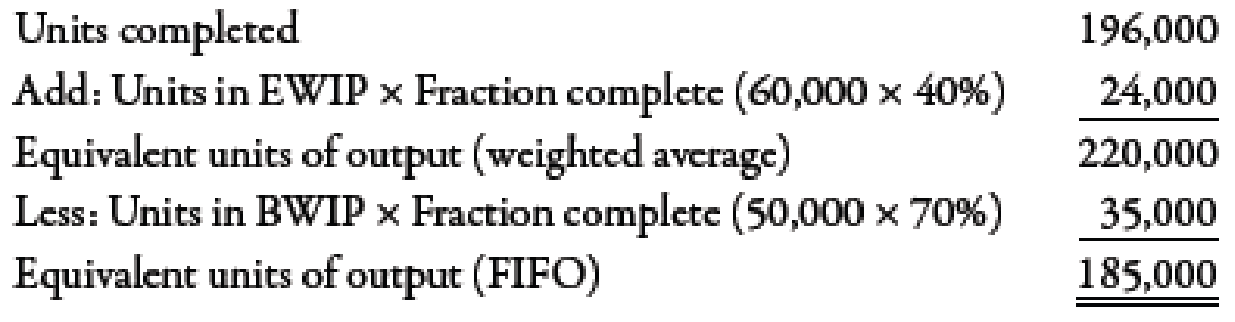# Weighted Average Method, Unit Costs, Valuing Inventories Byford Inc. produces a product that passes through two processes. During November, equivalent units were calculated using the weighted average method: The costs that Byford had to account for during the month of November were as follows: Required: 1. Using the weighted average method, determine unit cost. 2. Under the weighted average method, what is the total cost of units transferred out? What is the cost assigned to units in ending inventory? 3. CONCEPTUAL CONNECTION Bill Johnson, the manager of Byford, is considering switching from weighted average to FIFO. Explain the key differences between the two approaches and make a recommendation to Bill about which method should be used.### Managerial Accounting: The Corners...

7th Edition
Maryanne M. Mowen + 2 others
Publisher: Cengage Learning
ISBN: 9781337115773### Managerial Accounting: The Corners...

7th Edition
Maryanne M. Mowen + 2 others
Publisher: Cengage Learning
ISBN: 9781337115773

#### Solutions

Chapter
Section
Chapter 6, Problem 46E
Textbook Problem
224 views

## Weighted Average Method, Unit Costs, Valuing InventoriesByford Inc. produces a product that passes through two processes. During November, equivalent units were calculated using the weighted average method:The costs that Byford had to account for during the month of November were as follows:Required: 1. Using the weighted average method, determine unit cost. 2. Under the weighted average method, what is the total cost of units transferred out? What is the cost assigned to units in ending inventory? 3. CONCEPTUAL CONNECTION Bill Johnson, the manager of Byford, is considering switching from weighted average to FIFO. Explain the key differences between the two approaches and make a recommendation to Bill about which method should be used.

Expert Solution

1.

To determine

Calculate unit cost by using weighted average method.

### Explanation of Solution

Weighted Average Method:

The method in which both prior cost and cost incurred during the period are added to calculate unit cost is known as weighted average method. In this method, to calculate unit cost, summation of cost of BWIP and cost added during period is divided by equivalent units.

Use the following formula to calculate unit cost:

Unit cost=Cost of BWIP+Costs added during month

Expert Solution

2.

To determine

Compute total cost of goods transferred out and cost of EWIP.

Expert Solution

3.

To determine

Describe the difference between weighted average and FIFO and suggest Person B about which method should be used.

### Want to see the full answer?

Check out a sample textbook solution.See solution

### Want to see this answer and more?

Bartleby provides explanations to thousands of textbook problems written by our experts, many with advanced degrees!

See solution